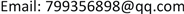1. 引言

2. 梯度下降法

2.1. 梯度下降(GD)算法

2.2. 梯度下降法的延伸

θ i = θ i − α ∗ ∂ J ( θ ) ∂ θ i = θ i − α ∗ ∑ j = 0 m ( y j − h θ ( x j ) ) x j i (1)

θ i = θ i − α ∗ ∂ J ( θ ) ∂ θ i = θ i − α ∗ ( y j − h θ ( x j ) ) x j i (2)

θ i = θ i − α ∗ ∑ j = t t + x − 1 ( y j − h θ ( x j ) ) x j i (3)

2.3. 加入动量的随机梯度下降

1964年Poljak提出了经典的动量方法  ，增加了动量矢量v，它积累了历史梯度方向，来代替了真正的梯度。速度是以动量参数 α 的衰减力度进行更新，若动量参数 α 越大，则之前的梯度对现在方向的影响也越大。直观的讲，若当前时刻的梯度与历史时刻梯度方向相似，这种趋势会在当前时刻加强，若方向相反，则当前的梯度方向趋势会减弱。

2.5. RMSProp

Nesterv算法可加速梯度，故而将Nesterv加入到RMSProp  ，这样既改变了学习率，Nesterov引入动量又改变了梯度，从两方面对参数进行了更新。

2014年，ADAM  算法被Kingma等人提出，它是moment与RMSProp的结合，Adam 不仅如RMSProp算法那样基于一阶矩均值计算适应性参数学习率，它同时还充分利用了梯度的二阶矩均值(即有偏方差)，适合解决含大规模的数据和参数的优化目标，也适合解决包含高噪声或稀疏梯度的问题。具体来说，算法计算了梯度的指数移动均值，超参数 ρ 1 和 ρ 2 控制了这些移动均值的衰减率。 ρ 1 和 ρ 2 的默认参数值接近于1，因此矩估计的偏差接近于0。该偏差通过首先计算带偏差的估计而后计算偏差修正后的估计而得到提升。

Continued

2.9. ANGD

Continued

3. 学习率衰减

3.1. 分段常数衰减

Parameter descriptio

learning_rate初始学习率
global_step用于衰减计算的全局步数，非负，用于逐步计算衰减指数
decay_steps衰减步数，必须是正值，决定衰减周期
decay_rate衰减率
end_learning_rate最低的最终学习率
cycle学习率下降后是否重新上升
alpha最小学习率
num_periods衰减余弦部分的周期数
initial_variance噪声的初始方差
variance_decay衰减噪声的方差

3.2. 指数衰减

decayed_learning_rate = learning_rate ∗ decay_rate global_step decay_steps (4)

3.3. 自然指数衰减

decayed_learning_rate = learning_rate ∗ exp − decay_rate ∗ global_step (5)

3.4. 多项式衰减

global_step = min ( global_step , decay_steps ) (6)

decayed_learning_rate = ( learning_rate − end_learning_rate ) ∗ ( 1 − global_step decay_steps ) power       + end_learning_rate (7)

decay_steps = decay_steps ∗ ceil ( global_step decay_steps ) (8)

3.5. 余弦衰减

global_step = min ( global_step , decay_steps ) (9)

cosine_decay = 0.5 ∗ ( 1 + cos ( pi ∗ global_step decay_steps ) ) (10)

decayed = ( 1 − alpha ) ∗ cosine_decay + alpha (11)

decayed_learning_rate = learning_rate ∗ decayed (12)

global_step = min ( global_step , decay_steps ) (13)

linear_decay = decay_steps − global_step decay_steps (14)

cosine_decay = 0.5 ∗ ( 1 + cos ( pi ∗ 2 ∗ num_periods ∗ global_step decay_steps ) ) (15)

decayed = ( alpha + linear_decay ) ∗ cosine_decay + beta (16)

decayed_learning_rate = learning_rate ∗ decayed (17)

global_step = min ( global_step , decay_steps ) (18)

linear_decay = decay_steps − global_step decay_steps (19)

cosine_decay = 0.5 ∗ ( 1 + cos ( pi ∗ 2 ∗ num_periods ∗ global_step decay_steps ) ) (20)

decayed = ( alpha + linear_decay + eps_t ) ∗ cosine_decay + beta (21)

decayed_learning_rate = learning_rate ∗ decayed (22)

4. 展望

1) 深度学习一般都是基于大量数据下的训练，而且模型一般都是监督学习，在训练前期，需要大量的人力去标注样本，如何节省前期的训练成本也是现在需要考虑的问题。

2) 基于优化器方法的梳理，如何加快收敛速度、减少搜索中的震荡仍是下一步研究中需要考虑的问题。牛顿法是经典的方法，但是由于二阶导数(Hessian矩阵)在大数据量的样本下，计算开销很大，可以尝试协方差的方向来考虑这个问题，是一个新的研究点。